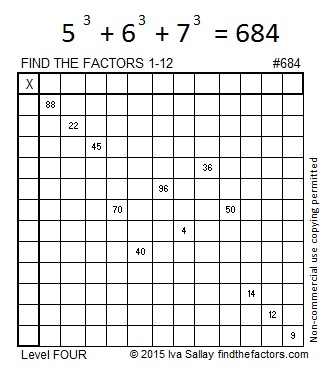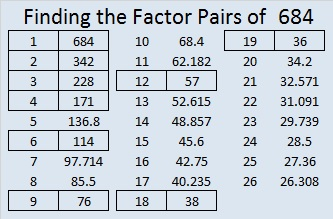# Consecutive Numbers That Add up to 684

684 is made from three consecutive even numbers so it is divisible by 3. The number in the middle of those consecutive numbers is divisible by 3 so 684 is also divisible by 9.

684 can be expressed as the sum of consecutive numbers in several ways:

• 227 + 228 + 229 = 684 because one of its factor pairs is 3 x 228.
• 72 + 73 + 74 + 75 + 76 + 77 + 78 + 79 + 80 = 684 because one of its factor pairs is 9 x 76.
• 82 + 83 + 84 + 85 + 86 + 87 + 88 + 89 = 684 because it is greater than 32, is divisible by 4 but not by 8, and 684/8 = 85 1/2.
• 27  + 28 + 29 + 30 + 31 + 32 + 33 + 34 + 35 + 36 + 37 + 38 + 39 + 40 + 41 + 42 + 43 + 44 + 45 = 684 because one of its factor pairs is 19 x 36.
• 17 + 18 + 19 + 20 + 21 + 22 + 23 + 24 + 25 + 26 + 27 + 28 + 29 + 30 + 31 + 32 + 33 + 34 + 35 + 36 + 37 + 38 + 39 + 40 = 684 because it is greater than 288, is divisible by 12 but not by 24, and 684/24 = 28 1/2.

684 is the sum of consecutive prime numbers 337 and 347.

684 is also the sum of the sixteen prime numbers from 13 to 73. Can you list all those prime numbers?

Best of all, the cubes of consecutive numbers 5, 6, and 7 add up to 684.Print the puzzles or type the solution on this excel file: 12 Factors 2015-11-16

—————————————————————————————————

• 684 is a composite number.
• Prime factorization: 684 = 2 x 2 x 3 x 3 x 19, which can be written 684 = (2^2) x (3^2) x 19
• The exponents in the prime factorization are 2, 2 and 1. Adding one to each and multiplying we get (2 + 1)(2 + 1)(1 + 1) = 3 x 3 x 2 = 18. Therefore 684 has exactly 18 factors.
• Factors of 684: 1, 2, 3, 4, 6, 9, 12, 18, 19, 36, 38, 57, 76, 114, 171, 228, 342, 684
• Factor pairs: 684 = 1 x 684, 2 x 342, 3 x 228, 4 x 171, 6 x 114, 9 x 76, 12 x 57, 18 x 38 or 19 x 36
• Taking the factor pair with the largest square number factor, we get √684 = (√36)(√19) = 6√19 ≈ 26.15339.—————————————————————————————————This site uses Akismet to reduce spam. Learn how your comment data is processed.